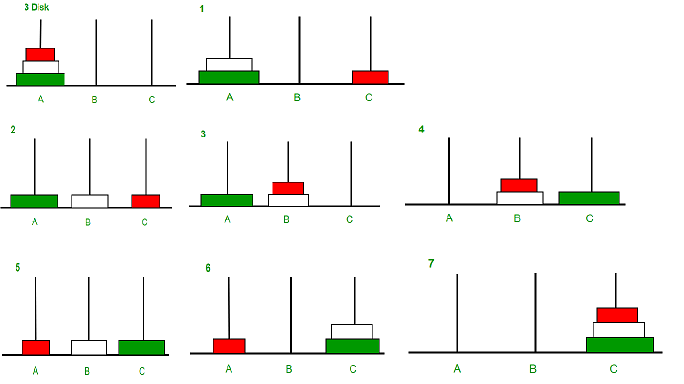# Program for Tower of Hanoi

• Difficulty Level : Medium
• Last Updated : 13 Jul, 2022

Tower of Hanoi is a mathematical puzzle where we have three rods and n disks. The objective of the puzzle is to move the entire stack to another rod, obeying the following simple rules:

1. Only one disk can be moved at a time.
2. Each move consists of taking the upper disk from one of the stacks and placing it on top of another stack i.e. a disk can only be moved if it is the uppermost disk on a stack.
3. No disk may be placed on top of a smaller disk.

Approach :```Let rod 1 = 'A', rod 2 = 'B', rod 3 = 'C'.
An example with 2 disks :
Step 1 : Shift first disk from 'A' to 'B'.

Step 2 : Shift second disk from 'A' to 'C'.

Step 3 : Shift first disk from 'B' to 'C'.```
```An example with 3 disks :
Step 1 : Shift first disk from 'A' to 'C'.
Step 2 : Shift second disk from 'A' to 'B'.
Step 3 : Shift first disk from 'C' to 'B'.

Step 4 : Shift third disk from 'A' to 'C'.

Step 5 : Shift first disk from 'B' to 'A'.
Step 6 : Shift second disk from 'B' to 'C'.
Step 7 : Shift first disk from 'A' to 'C'.
(Notice the gaps)```
```The pattern here is :
- Shift 'n-1' disks from 'A' to 'B', using C.
- Shift last disk from 'A' to 'C'.
- Shift 'n-1' disks from 'B' to 'C', using A.```Image illustration for 3 disks

Examples:

```Input : 2
Output : Disk 1 moved from A to B
Disk 2 moved from A to C
Disk 1 moved from B to C

Input : 3
Output : Disk 1 moved from A to C
Disk 2 moved from A to B
Disk 1 moved from C to B
Disk 3 moved from A to C
Disk 1 moved from B to A
Disk 2 moved from B to C
Disk 1 moved from A to C```
Recommended Practice

Implementation:

## C++

 `// C++ recursive function to ``// solve tower of hanoi puzzle ``#include ``using` `namespace` `std;`` ` `void` `towerOfHanoi(``int` `n, ``char` `from_rod,``                    ``char` `to_rod, ``char` `aux_rod) ``{ ``    ``if` `(n == 0) ``    ``{``        ``return``; ``    ``} ``    ``towerOfHanoi(n - 1, from_rod, aux_rod, to_rod); ``    ``cout << ``"Move disk "` `<< n << ``" from rod "` `<< from_rod <<``                                ``" to rod "` `<< to_rod << endl; ``    ``towerOfHanoi(n - 1, aux_rod, to_rod, from_rod); ``} `` ` `// Driver code``int` `main() ``{ ``    ``int` `n = 4; ``// Number of disks ``    ``towerOfHanoi(n, ``'A'``, ``'C'``, ``'B'``); ``// A, B and C are names of rods ``    ``return` `0; ``} `` ` `// This is code is contributed by rathbhupendra`

## Java

 `// JAVA recursive function to ``// solve tower of hanoi puzzle ``import` `java.util.*;``import` `java.io.*;``import` `java.math.*;``class` `GFG``{``static` `void` `towerOfHanoi(``int` `n, ``char` `from_rod,``                    ``char` `to_rod, ``char` `aux_rod) ``{ ``    ``if` `(n == ``0``) ``    ``{``        ``return``; ``    ``} ``    ``towerOfHanoi(n - ``1``, from_rod, aux_rod, to_rod); ``    ``System.out.println(``"Move disk "``+ n + ``" from rod "` `+ ``                       ``from_rod +``" to rod "` `+ to_rod ); ``    ``towerOfHanoi(n - ``1``, aux_rod, to_rod, from_rod); ``} `` ` `// Driver code``public` `static` `void`  `main(String args[]) ``{ ``    ``int` `n = ``4``; ``// Number of disks ``    ``towerOfHanoi(n, ``'A'``, ``'C'``, ``'B'``); ``// A, B and C are names of rods ``} ``}`` ` `// This code is contributed by jyoti369`

## Python3

 `# Recursive Python function to solve tower of hanoi`` ` `def` `TowerOfHanoi(n , from_rod, to_rod, aux_rod):``    ``if` `n ``=``=` `0``:``        ``return``    ``TowerOfHanoi(n``-``1``, from_rod, aux_rod, to_rod)``    ``print``(``"Move disk"``,n,``"from rod"``,from_rod,``"to rod"``,to_rod)``    ``TowerOfHanoi(n``-``1``, aux_rod, to_rod, from_rod)``         ` `# Driver code``n ``=` `4``TowerOfHanoi(n, ``'A'``, ``'C'``, ``'B'``) ``# A, C, B are the name of rods`` ` `# Contributed By Harshit Agrawal`

## C#

 `// C# recursive program to solve tower of hanoi puzzle``using` `System;``class` `GFG``{``    ``static` `void` `towerOfHanoi(``int` `n, ``char` `from_rod, ``char` `to_rod, ``char` `aux_rod)``    ``{``        ``if` `(n == 0)``        ``{``            ``return``;``        ``}``        ``towerOfHanoi(n-1, from_rod, aux_rod, to_rod);``        ``Console.WriteLine(``"Move disk "` `+ n + ``" from rod "` `+  ``                          ``from_rod + ``" to rod "` `+ to_rod);``        ``towerOfHanoi(n-1, aux_rod, to_rod, from_rod);``    ``}``     ` `    ``//  Driver method``    ``public` `static` `void` `Main(String []args)``    ``{``        ``int` `n = 4; ``// Number of disks``        ``towerOfHanoi(n, ``'A'``, ``'C'``, ``'B'``);  ``// A, B and C are names of rods``    ``}``}`` ` `//This code is contributed by shivanisinghss2110`

## PHP

 ` 0){``        ``TOH(``\$n``-1, ``\$A``, ``\$C``, ``\$B``);``        ``echo` `"Move disk from rod \$A to rod \$C \n"``;``        ``move(``\$A``, ``\$C``);``        ``dispPoles();``        ``TOH(``\$n``-1, ``\$B``, ``\$A``, ``\$C``);``    ``}``    ``else` `{``        ``return``;``    ``}``}`` ` `function` `initPoles(``\$n``){``    ``global` `\$poles``;`` ` `    ``for` `(``\$i``=``\$n``; ``\$i``>=1; --``\$i``){``        ``\$poles``[] = ``\$i``;``    ``}``}`` ` ` ` `function` `move(``\$source``, ``\$destination``){``    ``global` `\$poles``;``     ` `    ``// get source and destination pointers``    ``if` `(``\$source``==``"A"``) ``\$ptr1``=0;``    ``elseif` `(``\$source``==``"B"``) ``\$ptr1` `= 1;``    ``else` `\$ptr1` `= 2;``     ` `    ``if` `(``\$destination``==``"A"``) ``\$ptr2` `= 0;``    ``elseif` `(``\$destination``==``"B"``) ``\$ptr2` `= 1;``    ``else` `\$ptr2` `= 2;``     ` `    ``\$top` `= ``array_pop``(``\$poles``[``\$ptr1``]);``    ``array_push``(``\$poles``[``\$ptr2``], ``\$top``);``}`` ` `function` `dispPoles(){  ``    ``global` `\$poles``;``    ``echo` `"A: ["``.implode(``", "``, ``\$poles``).``"] "``;``    ``echo` `"B: ["``.implode(``", "``, ``\$poles``).``"] "``;``    ``echo` `"C: ["``.implode(``", "``, ``\$poles``).``"] "``;``    ``echo` `"\n\n"``;``}`` ` `\$numdisks` `= 4;``initPoles(``\$numdisks``);``echo` `"Tower of Hanoi Solution for \$numdisks disks: \n\n"``;``dispPoles();``TOH(``\$numdisks``);`` ` `// This code is contributed by ShreyakChakraborty``?>`

## Javascript

 ``

Output

```Move disk 1 from rod A to rod B
Move disk 2 from rod A to rod C
Move disk 1 from rod B to rod C
Move disk 3 from rod A to rod B
Move disk 1 from rod C to rod A
Move disk 2 from rod C to rod B
Move disk 1 from rod A to rod B
Move disk 4 from rod A to rod C
Move disk 1 from rod B to rod C
Move disk 2 from rod B to rod A
Move disk 1 from rod C to rod A
Move disk 3 from rod B to rod C
Move disk 1 from rod A to rod B
Move disk 2 from rod A to rod C
Move disk 1 from rod B to rod C```

Time complexity: O(2^n)
Space complexity: O(n)

Related Articles

My Personal Notes arrow_drop_up